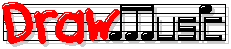Pop Music Theory

Lessons

Detailed Contents

Get Future Lessons

Detailed Contents

# Lesson 22: Diatonic Triads

This lesson teaches diatonic triads, which are important for understanding the chord progressions used in many songs (or writing your own songs).

Before taking this lesson, you should know:

Diatonic means "staying within the key". So, for example, the diatonic triads in the key of B♭ major are the triads we can create using only pitches in the B♭ major scale.

There are just six "common" diatonic triads in any particular major key; we build these six triads on scale degrees 1 through 6 of the key's major scale. (We can also build a triad on degree 7, but that one is much less common, so I'm skipping it for now.) Let's find each of these six diatonic triads, one at a time:

## The I chord ("one-major")

Our first diatonic triad uses degrees 1 3 5 of our (example) B♭ major scale:
 1 2 3 4 5 6 7 8 B♭ C D E♭ F G A B♭
So, the pitches in this chord are B♭ D F; hopefully you recognize that this is a major triad (chord symbol: B♭); so our first triad's Roman symbol is just I ("one major").

## The IIm chord ("two-minor")

The second triad uses degrees 2 4 6 of our B♭ major scale:
 1 2 3 4 5 6 7 8 B♭ C D E♭ F G A B♭
So, the pitches in this chord are C E♭ G; this is a minor triad (chord symbol: Cm); so the Roman symbol is IIm ("two minor").

## The IIIm chord ("three-minor")

The third triad uses degrees 3 5 7 of our B♭ major scale:
 1 2 3 4 5 6 7 8 B♭ C D E♭ F G A B♭
So, the pitches in this chord are D F A; this is a minor triad (chord symbol: Dm); so the Roman symbol is IIIm ("three minor").

## The IV chord ("four-major")

The fourth triad uses degrees 4 6 8 of our B♭ major scale:
 1 2 3 4 5 6 7 8 B♭ C D E♭ F G A B♭
So, the pitches in this chord are E♭ G B♭; this is a major triad (chord symbol: E♭); so the Roman symbol is IV ("four major").

## The V chord ("five-major")

The fifth triad uses degrees 5 7 9 of our B♭ major scale:
 1 2 3 4 5 6 7 8 9 10 B♭ C D E♭ F G A B♭ C D
So, the pitches in this chord are F A C; this is a major triad (chord symbol: F); so the Roman symbol is V ("five major").

## The VIm chord ("six-minor")

Finally, the sixth triad uses degrees 6 8 10 of our B♭ major scale:
 1 2 3 4 5 6 7 8 9 10 B♭ C D E♭ F G A B♭ C D
So, the pitches in this chord are G B♭ D; this is a minor triad (chord symbol: Gm); so the Roman symbol is VIm ("six minor").

## Summing Up

Putting it all together, in Roman chord symbols, these are the common major-key diatonic triads:

## I   IIm   IIIm   IV   V   VIm

And, for reference, here are they are as "specific" chords, in the common major keys:

 Key I IIm IIIm IV V VIm A♭ major A♭ B♭m Cm D♭ E♭ Fm A major A Bm C♯m D E F♯m B♭ major B♭ Cm Dm E♭ F Gm B major B C♯m D♯m E F♯ G♯m C major C Dm Em F G Am D♭ major D♭ E♭m Fm G♭ A♭ B♭m D major D Em F♯m G A Bm E♭ major E♭ Fm Gm A♭ B♭ Cm E major E F♯m G♯m A B C♯m F major F Gm Am B♭ C Dm F♯ major F♯ G♯m A♯m B C♯ D♯m G♭ major G♭ A♭m B♭m C♭ D♭ E♭m G major G Am Bm C D Em

When you understand these diatonic triads:
• You can get suggestions for "practice activities" to help you learn to use them in the next lesson, Lesson 23: Using Diatonics;
• Or, if you don't need practice suggestions, you can jump to learning about the different effects of the different diatonic triads in Lesson 24: Tonic Function.